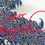# Two-Dimensional Kinematics: Motion in a Plane

First Step: Choosing Coordinates

Kinematics involves displacement, velocity, and acceleration which are all vector quantities that require both a magnitude and direction.

Therefore, to begin a problem in two-dimensional kinematics you must first define the coordinate system you are using. Generally it will be in terms of an x-axis and a y-axis, oriented so that the motion is in the positive direction, although there may be some circumstances where this is not the best method.

In cases where gravity is being considered, it is customary to make the direction of gravity in the negative-y direction. This is a convention that generally simplifies the problem, although it would be possible to perform the calculations with a different orientation if you really desired.

Velocity Vector

The position vector r is a vector that goes from the origin of the coordinate system to a given point in the system. The change in position (Δ r , pronounced "Delta r") is the difference between the start point ( r 1) to end point ( r 2). We define the average velocity ( v av) as: vav = (r2 - r1) / (t2 - t1) = Δr/Δt Taking the limit as Δt approaches 0, we achieve the instantaneous velocity v. In calculus terms, this is the derivative of r with respect to t, or dr/dt. As the difference in time reduces, the start and end points move closer together. Since the direction of r is the same direction as v, it becomes clear that the instantaneous velocity vector at every point along the path is tangent to the path.

Velocity Components

The useful trait of vector quantities is that they can be broken up into their component vectors. The derivative of a vector is the sum of its component derivatives, therefore: vx = dx/dt vy = dy/dt The magnitude of the velocity vector is given by the Pythagorean Theorem in the form: |v| = v = sqrt (vx2 + vy2) The direction of v is oriented alpha degrees counter-clockwise from the x-component, and can be calculated from the following equation: tan alpha = vy / vx Acceleration Vector

Acceleration is the change of velocity over a given period of time. Similar to the analysis above, we find that it's Δv/Δt. The limit of this as Δt approaches 0 yields the derivative of v with respect to t. In terms of components, the acceleration vector can be written as:

ax = dvx/dt ay = dvy/dt or

ax = d2x/dt2 ay = d2y/dt2

The magnitude and angle (denoted as beta to distinguish from alpha) of the net acceleration vector are calculated with components in a fashion similar to those for velocity. Working with Components

Frequently, two-dimensional kinematics involves breaking the relevant vectors into their x- and y-components, then analyzing each of the components as if they were one-dimensional cases. Once this analysis is complete, the components of velocity and/or acceleration are then combined back together to obtain the resulting two-dimensional velocity and/or acceleration vectors. Three-Dimensional Kinematics

The above equations can all be expanded for motion in three dimensions by adding a z-component to the analysis. This is generally fairly intuitive, although some care must be made in making sure that this is done in the proper format, especially in regards to calculating the vector's angle of orientation.Note by Madison Jones
5 years, 10 months ago

This discussion board is a place to discuss our Daily Challenges and the math and science related to those challenges. Explanations are more than just a solution — they should explain the steps and thinking strategies that you used to obtain the solution. Comments should further the discussion of math and science.

When posting on Brilliant:

• Use the emojis to react to an explanation, whether you're congratulating a job well done , or just really confused .
• Ask specific questions about the challenge or the steps in somebody's explanation. Well-posed questions can add a lot to the discussion, but posting "I don't understand!" doesn't help anyone.
• Try to contribute something new to the discussion, whether it is an extension, generalization or other idea related to the challenge.
• Stay on topic — we're all here to learn more about math and science, not to hear about your favorite get-rich-quick scheme or current world events.

MarkdownAppears as
*italics* or _italics_ italics
**bold** or __bold__ bold
- bulleted- list
• bulleted
• list
1. numbered2. list
1. numbered
2. list
Note: you must add a full line of space before and after lists for them to show up correctly
paragraph 1paragraph 2

paragraph 1

paragraph 2

[example link](https://brilliant.org)example link
> This is a quote
This is a quote
    # I indented these lines
# 4 spaces, and now they show
# up as a code block.

print "hello world"
# I indented these lines
# 4 spaces, and now they show
# up as a code block.

print "hello world"
MathAppears as
Remember to wrap math in $$ ... $$ or $ ... $ to ensure proper formatting.
2 \times 3 $2 \times 3$
2^{34} $2^{34}$
a_{i-1} $a_{i-1}$
\frac{2}{3} $\frac{2}{3}$
\sqrt{2} $\sqrt{2}$
\sum_{i=1}^3 $\sum_{i=1}^3$
\sin \theta $\sin \theta$
\boxed{123} $\boxed{123}$

## Comments

Sort by:

Top Newest

Important reminders.

Try adding them to the wikis.

:)

- 5 years, 10 months ago

Log in to reply

×

Problem Loading...

Note Loading...

Set Loading...